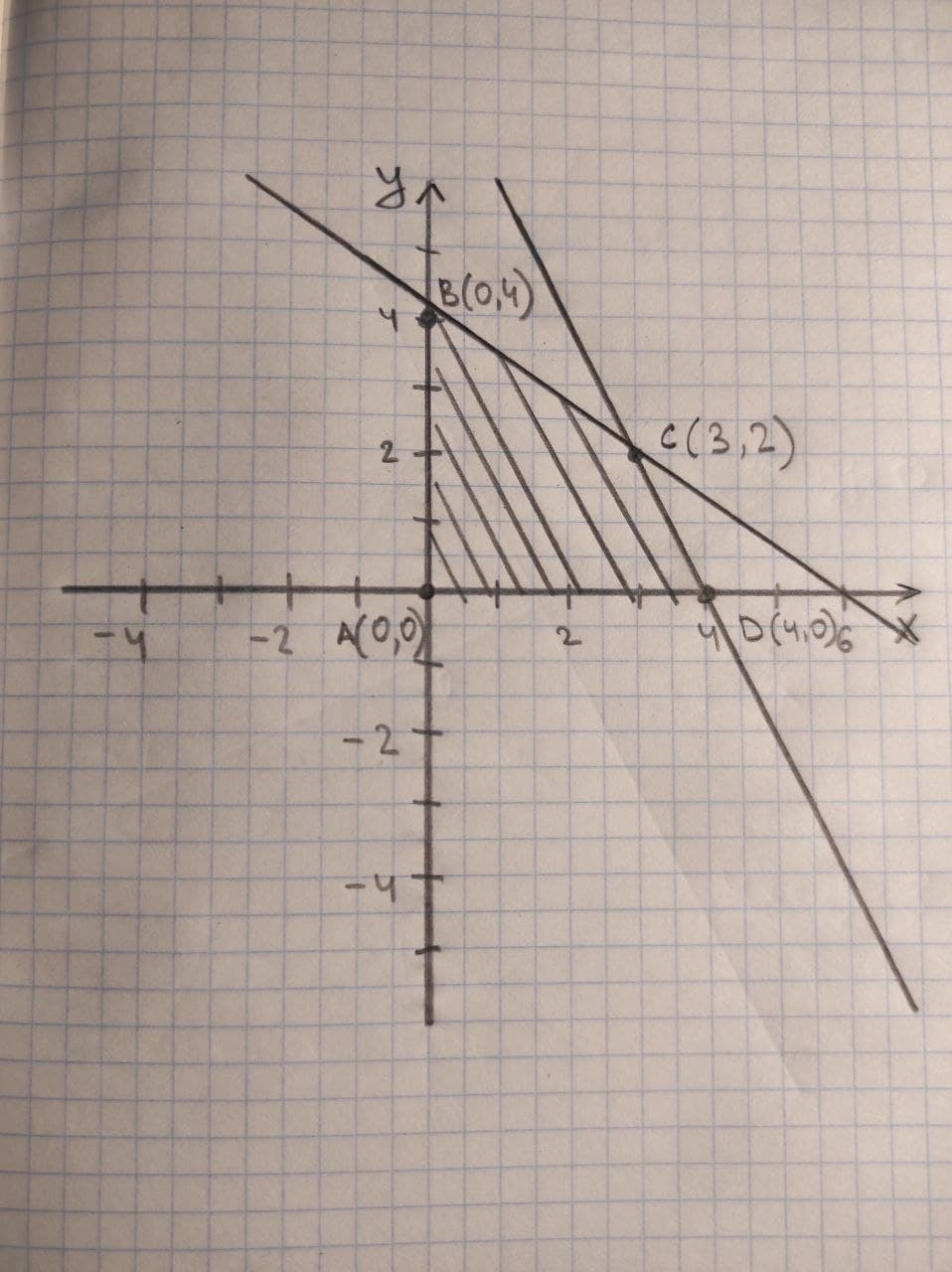# An objective function and a system of linear inequalities representing constraints are given. a. Graph the system of inequalities representing the conArmorikam 2021-01-02 Answered
An objective function and a system of linear inequalities representing constraints are given.
a. Graph the system of inequalities representing the constraints.
b. Find the value of the objective function at each corner of the graphed region.
c. Use the values in part (b) to determine the maximum value of the objective function and the values of x and y for which the maximum occurs.
$z=2x+3y$
$\left\{\begin{array}{c}x\phantom{\rule{1em}{0ex}}\text{and}\phantom{\rule{1em}{0ex}}y\ge 0\\ 2x+y\le 8\\ 2x+3y\le 12\end{array}$
You can still ask an expert for help

## Want to know more about Inequalities systems and graphs?

• Questions are typically answered in as fast as 30 minutes

Solve your problem for the price of one coffee

• Math expert for every subject
• Pay only if we can solve itwornoutwomanC
$x\ge 0$ (1)
$y\ge 0$ (2)
$2x+y\le 8$ (3)
$2x+3y\le 12$ (4)
a) Graph of given inequalities
b) The value at corner is given in graph.
c) find the maximum at which corner,
At point A (0,0)
z=2(0)+3(0) = 0
At point B (0,4)
z=2(0)+3(4) = 12
At point C (3,2)
z=2(3)+3(2) = 12
At point D (4,0)
z=2(4)+3(0) = 8